Test: Introduction to Signals

# Test: Introduction to Signals

Test Description

## 10 Questions MCQ Test GATE Electrical Engineering (EE) 2023 Mock Test Series | Test: Introduction to Signals

Test: Introduction to Signals for Electronics and Communication Engineering (ECE) 2023 is part of GATE Electrical Engineering (EE) 2023 Mock Test Series preparation. The Test: Introduction to Signals questions and answers have been prepared according to the Electronics and Communication Engineering (ECE) exam syllabus.The Test: Introduction to Signals MCQs are made for Electronics and Communication Engineering (ECE) 2023 Exam. Find important definitions, questions, notes, meanings, examples, exercises, MCQs and online tests for Test: Introduction to Signals below.
Solutions of Test: Introduction to Signals questions in English are available as part of our GATE Electrical Engineering (EE) 2023 Mock Test Series for Electronics and Communication Engineering (ECE) & Test: Introduction to Signals solutions in Hindi for GATE Electrical Engineering (EE) 2023 Mock Test Series course. Download more important topics, notes, lectures and mock test series for Electronics and Communication Engineering (ECE) Exam by signing up for free. Attempt Test: Introduction to Signals | 10 questions in 30 minutes | Mock test for Electronics and Communication Engineering (ECE) preparation | Free important questions MCQ to study GATE Electrical Engineering (EE) 2023 Mock Test Series for Electronics and Communication Engineering (ECE) Exam | Download free PDF with solutions
 1 Crore+ students have signed up on EduRev. Have you?
Test: Introduction to Signals - Question 1

### The signal x(t) = A cos(ωt + φ) is:

Detailed Solution for Test: Introduction to Signals - Question 1

All bounded periodic signals are power signals, because they do not converge to a finite value so their energy is infinite and their power is finite.

Test: Introduction to Signals - Question 2

### What is the total energy of the rectangular pulse shown in figure below?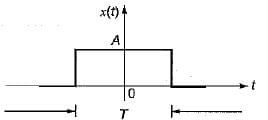Detailed Solution for Test: Introduction to Signals - Question 2Test: Introduction to Signals - Question 3

### The auto-correlation function Rx(τ) satisfies which one of the following properties?

Detailed Solution for Test: Introduction to Signals - Question 3

The autocorrelation function of a signal is defined as the measure of similarity or coherence between a signal and its time delayed version. Thus, autocorrelation is the correlation of a signal with itself.

Property of autocorrelation function:

• The autocorrelation function of energy signals exhibits complex conjugate symmetry, which means the real part of autocorrelation function R(τ) is an even function of delay parameter ( τ) and the imaginary part of R(τ) is an odd function of the parameter τ. Thus,

R(τ)=R∗(−τ)

Test: Introduction to Signals - Question 4

The auto-correlation function Rx(τ) of the signal x(t) = V sinωt is given by:

Detailed Solution for Test: Introduction to Signals - Question 4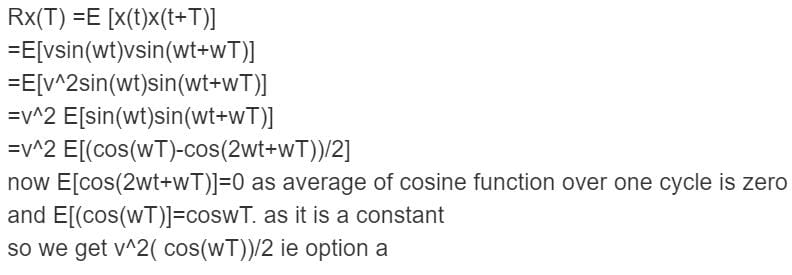Test: Introduction to Signals - Question 5

The sampling of a function f(t) = sin(2πf0t) starts from zero-crossing. The signal can be detected, if sampling time T is:

Detailed Solution for Test: Introduction to Signals - Question 5

Because fs ≤ 2f0,

Ts ≤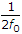.

Test: Introduction to Signals - Question 6

What is the power and energy of the unit step sequence u(n) respectively?

Detailed Solution for Test: Introduction to Signals - Question 6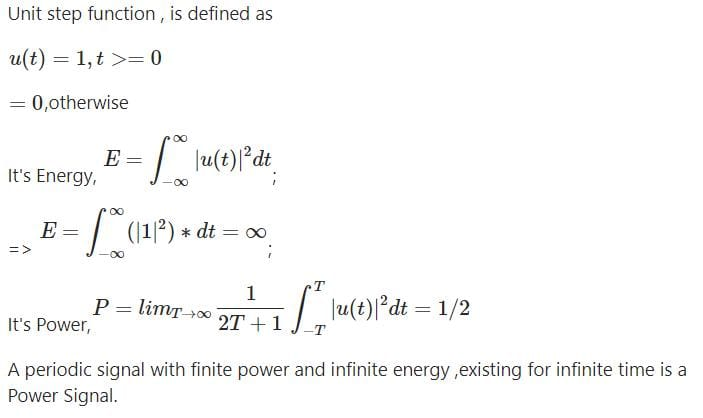Test: Introduction to Signals - Question 7

Let δ(f) is the delta function the value of integral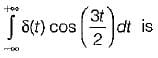Test: Introduction to Signals - Question 8

If a signal f(t) has energy ‘E’ the energy of the signal f(2t) is equal to:

Detailed Solution for Test: Introduction to Signals - Question 8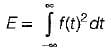and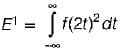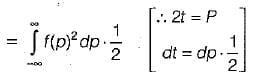⇒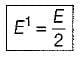Test: Introduction to Signals - Question 9

Consider the sequence: x[n] = [- 4 - j5, 1 + j2, 4], the conjugate anti-symmetric part of the sequence is:

Detailed Solution for Test: Introduction to Signals - Question 9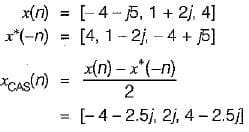Test: Introduction to Signals - Question 10

The function x(t) is shown in the figure. Even and odd parts of a unit step function u(t) are respectively: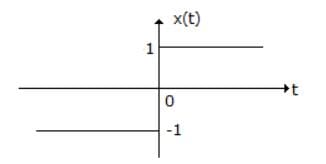Detailed Solution for Test: Introduction to Signals - Question 10

x(t) = u(t) - u(-t)

u(t) =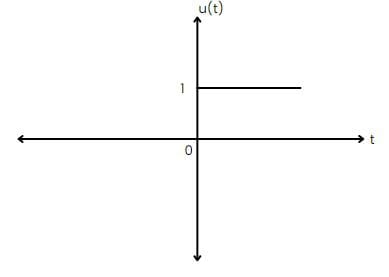u(-t) =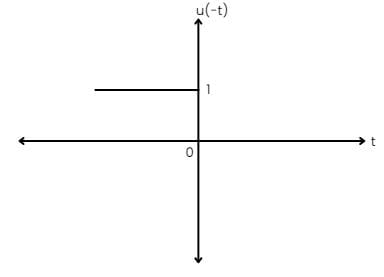-u(-t) =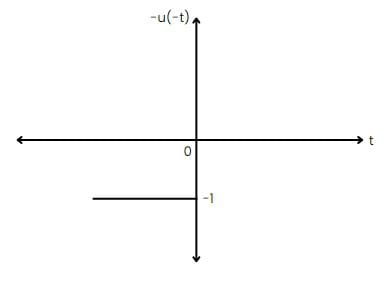Hence,

x(t) = u(t) - u(-t)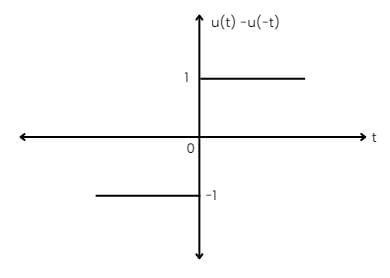even part = [u(t) + u(-t)] / 2 = 1/2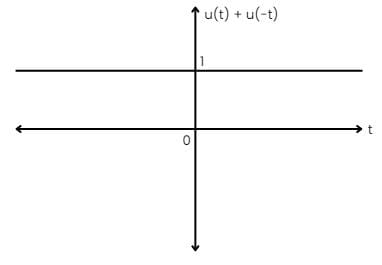odd part = [u(t) - u(-t)] / 2 = 1/2[x(t)]

## GATE Electrical Engineering (EE) 2023 Mock Test Series

22 docs|274 tests
Information about Test: Introduction to Signals Page
In this test you can find the Exam questions for Test: Introduction to Signals solved & explained in the simplest way possible. Besides giving Questions and answers for Test: Introduction to Signals, EduRev gives you an ample number of Online tests for practice

## GATE Electrical Engineering (EE) 2023 Mock Test Series

22 docs|274 tests(Scan QR code)